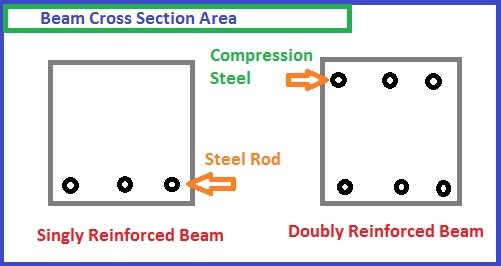# Singly reinforced beam design- Step by Step Numerical

The Concrete beam whose only tension zone of cross-section area is covered with steel rod is known as a singly reinforced beam.

A reinforced concrete (RC) has several members in the form of beams, columns, slabs, and walls that are rigidly connected to a form of a monolithic frame.

“Beams are members that are primarily subjected to flexure or bending and often support slabs.”

Beams support the loads applied to them by slabs and their own weight by internal moments and shears.

In a reinforced concrete beam of rectangular cross-section, if the reinforcement is provided only in the tension zone, it is called a singly reinforced rectangular beam.

In the Singly reinforced beam, the ultimate bending moment and the tension due to bending are carried by the reinforcement. While the compressions carried by the concrete.

Practically, it is not possible that the reinforcement in the tension zone only, because we need to tie the stirrups.

## Analysis Steps of Beams

Steps for the analysis of singly reinforced rectangular beams are as follows:

Step(1)

Calculate area of reinforcement steel (Ast)

Step(2)

Calculate depth of Neutral axis (xu) by using Horizontal equilibrium i.e. by using the expression.

Compressive force in concrete = Tensile force in steel

Or,  C  =  T

Or, 0.36fck bxu  = fs Ast

Step(3)

Calculate the limiting value of depth on neutral axis (xu,max) by using clause 38.1 of code IS 456:2000.

Step(4)

Compare xu with xu,max and determine whether the section is balanced section, under reinforced section or over reinforced section.

Step (5)

Find the moment of resistance (Mu) by using the expression for balanced and under reinforced section and expression for over reinforced section.

## Design of Singly reinforced beamSingly reinforced beam design

1) Design a reinforced concrete beam of size 300 mm X 700 mm is subjected to a bending moment of 150 KNm. Take effective cover as 40 mm. Use M20 concrete and Fe 415 steel.

Solution;

Given:-

Breadth (b) = 300 mm

Depth (d) = 700-40  = 660 mm

M = 150 KNm

Fck = 20 N/mm2  ,

fy = 415 N/mm2

Step-1

Step-2Calculate Limiting value of resistance,

Step-3

Calculate Designed moment

Step-4

Calculate Area of steel Required (Ast)

Read Also,

Doubly reinforced beam design- Step by Step Numerical

Design of compression members- Design of Column

Types of concrete mix design| mix ratio of cement-civil concept

Top 10 Quick guides for Reinforced concrete Column design

Step by Step procedure for Dog legged staircase design- With Example

error: Content is protected !!